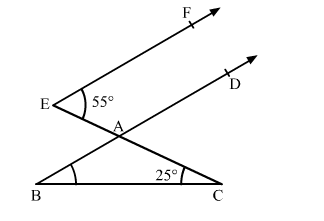# In the given figure, BAD || EF, ∠AEF = 55° and ∠ACB = 25°, find ∠ABC.

Question:

In the given figure, BAD || EF, ∠AEF = 55° and ∠ACB = 25°, find ∠ABC.Solution:

In the given figure, EF || BD and CE is the transversal.

∴ ∠CAD = ∠AEF         (Pair of corresponding angles)

In ∆ABC,

∠CAD = ∠ABC + ∠ACB       (Exterior angle of a triangle is equal to the sum of the two interior opposite angles)

⇒ 55° = ∠ABC + 25°

⇒ ∠ABC = 55° − 25° = 30°

Thus, the measure of ∠ABC is 30°.